Courses

# Ex 13.1 NCERT Solutions- Surface Areas and Volumes Class 10 Notes | EduRev

## Class 10 : Ex 13.1 NCERT Solutions- Surface Areas and Volumes Class 10 Notes | EduRev

The document Ex 13.1 NCERT Solutions- Surface Areas and Volumes Class 10 Notes | EduRev is a part of the Class 10 Course Class 10 Mathematics by VP Classes.
All you need of Class 10 at this link: Class 10

[Unless stated otherwise, take π = 22/7]

Q.1. Two cubes each of volume 64 cm3 are joined end to end. Find the surface area of the resulting cuboid.
Sol.  Volume of each cube = 64 cm3
∴ Total volume of the two cubes = 2 × 64 cm3 = 128 cm3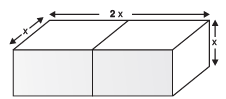Let the edge of each cube = x
∴ x3 = 64 = 43
⇒ x =4 cm
Now, Length of the resulting cuboid 'l' = 2x cm
Breadth of the resulting cuboid 'b' = x cm
Height of the resulting cuboid 'h' = x cm
∴ Surface area of the cuboid = 2 (lb + bh + hl)
= 2 [(2x · x) + (x · x) + (x · 2x)]
= 2 [(2 × 4 × 4) + (4 × 4) + (4 × 2 × 4)] cm2
= 2 [32 + 16 + 32] cm2 = 2  cm2 = 160 cm2.

Q.2. A vessel is in the form of a hollow hemisphere mounted by a hollow cylinder. The diameter of the hemisphere is 14 cm and the total height of the vessel is 13 cm. Find the inner surface area of the vessel.
Sol.

For the cylindrical part
Radius (r) = 7 cm
Height (h) = 6 cm
∴ Curved surface area
= 2πrh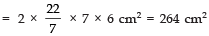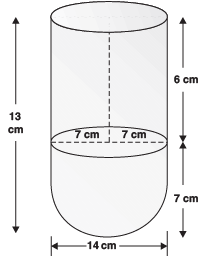For hemispherical part
Radius (r) = 7 cm
∴ Surface area = 2πr2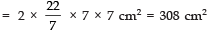∴ Total surface area
= (264 + 308) cm2 = 572 cm2.

Q.3. A toy is in the form of a cone of radius 3.5 cm mounted on a hemisphere of the same radius. The total height of the toy is 15.5 cm. Find the total surface area of the toy.
Sol. Here, r = 3.5 cm
∴ h = (15.5 − 3.5) cm = 12.0 cm
Surface area of the conical part = πrl
Surface area of the hemispherical part = 2πr2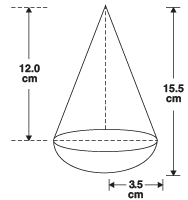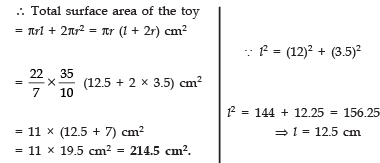Q.4. A cubical block of side 7 cm is surmounted by a hemisphere. What is the greatest diameter the hemisphere can have? Find the surface area of the solid.
Sol. Side of the block = 7 cm
⇒ The greatest diameter of the hemisphere = 7 cm
Surface area of the solid:
= [Total S.A. of the cubical block] + [S.A. of the hemisphere] − [Base area of the hemisphere]
= (6 × l2) + 2πr2 − πr2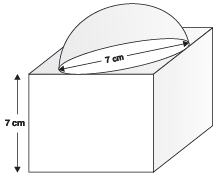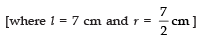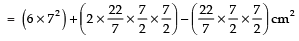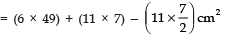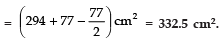Q.5. A hemispherical depression is cut out from one face of a cubical wooden block such that the diameter l of the hemisphere is equal to the edge of the cube. Determine the surface area of the remaining solid.
Sol. Let ‘l’ be the side of the cube.
∴ The greatest diameter of the curved hemisphere = l
⇒ Radius of the curved hemisphere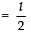∴ Surface area of hemisphere = 2πr2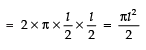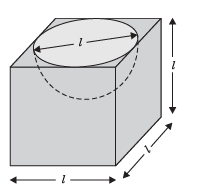Base area of the hemisphere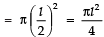Surface area of the cube = 6 × l2 = 6l2
∴ Surface area of the remaining solid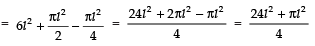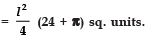Q.6. A medicine capsule is in the shape of a cylinder with two hemispheres stuck to each of its ends (see Fig.). The length of the entire capsule is 14 mm and the diameter of the capsule is 5 mm. Find its surface area.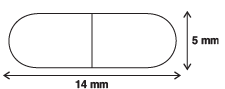Sol. Radius of the hemispherical part
= 5/2 mm = 2.5 mm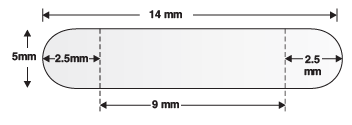∴ Surface area of one hemispherical part = 2πr2
⇒ Surface area of both hemispherical parts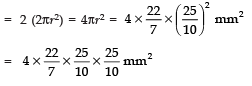Area of cylindrical part =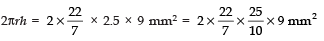∴ Total surface area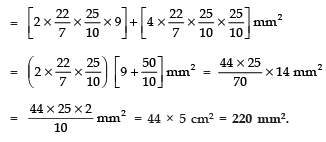Q.7. A tent is in the shape of a cylinder surmounted by a conical top. If the height and diameter of the cylindrical part are 2.1 m and 4 m respectively, and the slant height of the top is 2.8 m, find the area of the canvas used for making the tent. Also, find the cost of the canvas of the tent at the rate of Rs 500 per m2. (Note that the base of the tent will not be covered with canvas.)
Sol.

For cylindrical part:
Radius (r) = 4/2 m = 2 m
Height (h) = 2.1 m
∴ Curved surface area = 2πrh =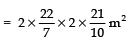For conical part:
Slant height (l) = 2.8 m
Base radius (r)= 2m
∴ Curved surface area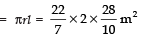Total surface area
= [Surface area of the cylindrical part] + [Surface area of conical part]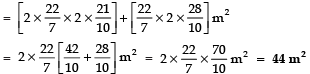Cost of the canvas used
Cost of 1 m2 of canvas = Rs 500
∴ Cost of 44 m2 of canvas = Rs 500 × 44 = Rs. 22000

Q 8. From a solid cylinder whose height is 2.4 cm and diameter 1.4 cm, a conical cavity of the same height and same diameter is hollowed out. Find the total surface area of the remaining solid to the nearest cm2.
Sol.

For cylindrical part:
Height = 2.4 cm
Diameter = 1.4 cm
⇒ Radius (r) = 0.7 cm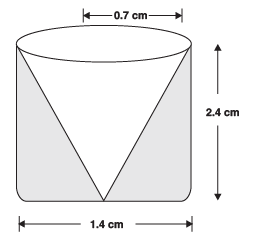For conical part:
Base area (r) = 0.7 cm
Height (h) = 2.4 cm
∴ Slant height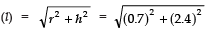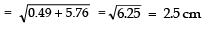∴ Curved surface area of the conical part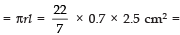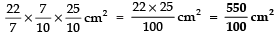Base area of the conical part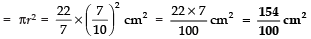Total surface area of the remaining solid: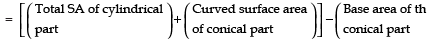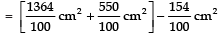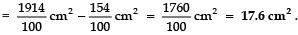Q 9. A wooden article was made by scooping out a hemisphere from each end of a solid cylinder, as shown in Fig. If the height of the cylinder is 10 cm, and its base is of radius 3.5 cm, find the total surface area of the article.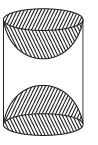Sol. Radius of the cylinder (r) = 3.5 cm
Height of the cylinder (h) = 10 cm
∴ Total surface area = 2πrh + 2πr2 = 2πr (h + r)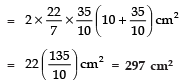Curved surface area of a hemisphere = 2πr2
Curved surface area of both hemispheres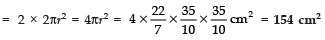Base area of a hemisphere = πr2
∴ Base area of both hemispheres = 2πr2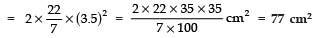∴ Total surface area of the remaining solid
= 297 cm2 + 154 cm2 − 77 cm2
= (451 − 77) cm2 = 374 cm2.

Offer running on EduRev: Apply code STAYHOME200 to get INR 200 off on our premium plan EduRev Infinity!

132 docs

,

,

,

,

,

,

,

,

,

,

,

,

,

,

,

,

,

,

,

,

,

;